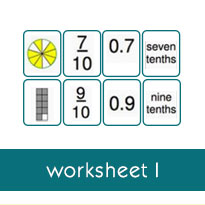# Converting a Proper Fraction With a Denominator of 2, 4, or 5 to a Decimal: Worksheets

Welcome to the Converting Fractions to Decimals section at Tutorialspoint.com. On this page, you will find worksheets on writing a fraction and a decimal for a shaded region of a figure, converting a fraction with a denominator of 10 or 100 or 1000 to a decimal, converting a fraction with a denominator of 2, 4 or 5 to a decimal, converting fractions and mixed numbers into terminating decimals and repeating decimals, using a calculator to convert a fraction to a rounded decimal, comparing and ordering fractions and decimals and so on.

To practice Math skills, there is nothing more effective than solving worksheets. Our free to download, printable worksheets help you practice Math concepts, and improve your analytical and problem-solving skills. We have 3 worksheets for each topic in the tutorial.